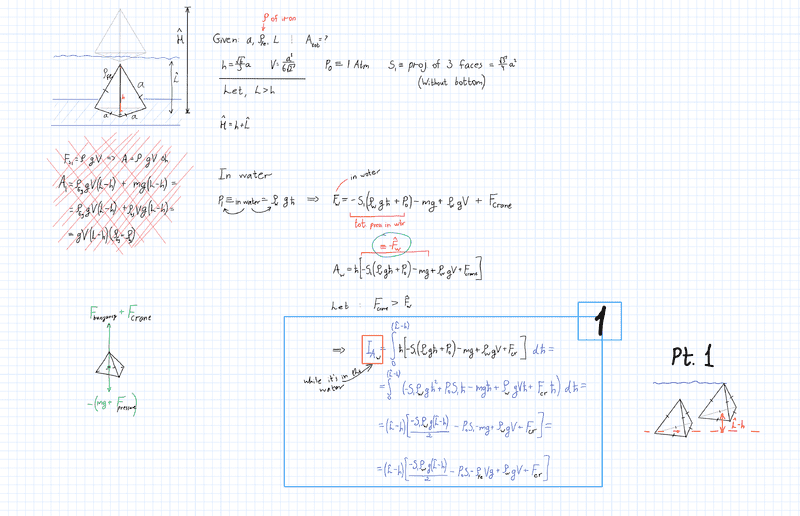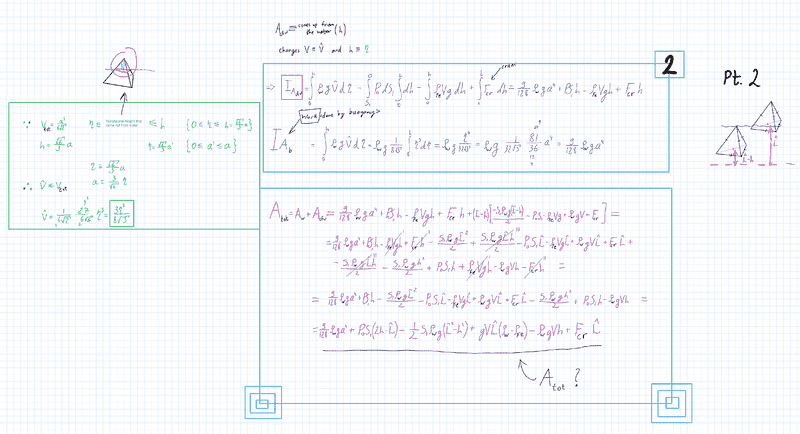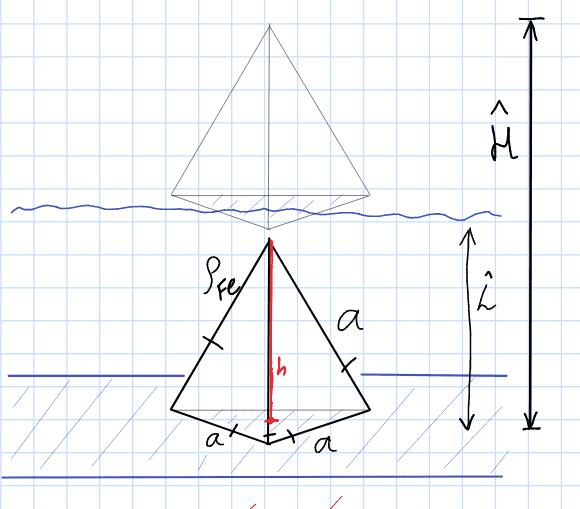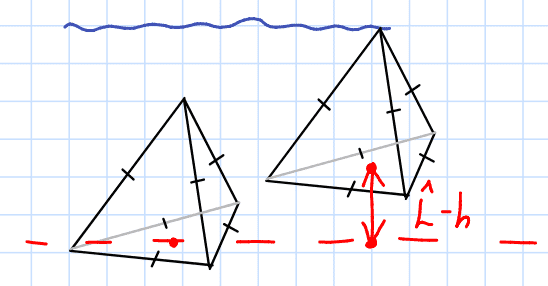# Work calculation for lifting a Tetrahedron-shaped object from the water

• NODARman
I did that, mg, buoyancy, pressure, and crane (imaginary) forces. The above statement suggests you may be ‘double counting’ the force of buoyancy.

#### NODARman

Homework Statement
.
Relevant Equations
.
Hi, I'm calculating the work done by regular tetrahedron during taking from the water by crane (USING INTEGRALS). I don't know how bad is that solved so if anyone checks my work and gives me some advice or hints I would be very glad.
Everything is written in the PDF file.
There were given:
Tetrahedron side - "a";
Water depth - "L";
The density of iron (tetrahedron material) - "rho (Fe)".

I assumed the density of water and the pulling force of the crane.

Also, I divide the problem into two parts, the first is the work done before it came out from the water, and the second one is starting to come out from it.

#### Attachments

• Calculate A.pdf
301.2 KB · Views: 57
Please remember that we do not support work shown only in attached images or PDFs. Please start to use LaTeX to post your work -- you will get more and better responses if you follow that rule here. See the LaTeX Guide link below the Edit window for more information.

BTW -- you can also generally turn in your assignments using LaTeX, so that way you don't have to do your work twice...•minimikanix
berkeman said:
Please remember that we do not support work shown only in attached images or PDFs. Please start to use LaTeX to post your work -- you will get more and better responses if you follow that rule here. See the LaTeX Guide link below the Edit window for more information.

BTW -- you can also generally turn in your assignments using LaTeX, so that way you don't have to do your work twice...Sorry, I'll edit the post. Thanks for the advice :)

•berkeman
berkeman said:
Please remember that we do not support work shown only in attached images or PDFs. Please start to use LaTeX to post your work -- you will get more and better responses if you follow that rule here. See the LaTeX Guide link below the Edit window for more information.

BTW -- you can also generally turn in your assignments using LaTeX, so that way you don't have to do your work twice...I have trouble with Latex input. Can you tell me how do I post or reply using that math input? I can't find it.NODARman said:
I have trouble with Latex input. Can you tell me how do I post or reply using that math input? I can't find it.Did you try https://www.physicsforums.com/help/latexhelp/?
But can you post the original question in full as an image, not a pdf?
Is it a solid tetrahedron of iron, initially resting with its base at depth L, fully submerged, then raised until just clear of the water?

haruspex said:
Did you try https://www.physicsforums.com/help/latexhelp/?
But can you post the original question in full as an image, not a pdf?
Yeah, I've tried that help page but, still couldn't do that.
Here're the images.•Lnewqban
I would just calculate the submerged volume of the tetrahedron as a function of height which would allow me to calculate the buoyancy. It seems like you are doing more than this.

Last edited:
Frabjous said:
I would just calculate the submerged volume of the tetrahedron as a function of height which would allow me to calculate the buoyancy. It seems like you are doing more than this.
Yes, the main question is to find the full work done by taking this object out from the water.

NODARman said:
Yes, the main question is to find the full work done by taking this object out from the water.
Don’t you then just have to integrate the sum of the force of gravity and buoyancy force?

Frabjous said:
Don’t you then just have to integrate the sum of the force of gravity and buoyancy force?
I did that, mg, buoyancy, pressure, and crane (imaginary) forces. I don't know if those are correct, that's why I'm posting.

There are three regions: 1) fully submerged, 2) partially submerged and 3) above surface. Let M,V be mass, volume of tetrahedron; h1, h2,h3 be distances lifted in each region. Buoyancy is technically an equilibrium phenomena so we are moving infinitely slowly.
Region 1) ##W_1=(M-\rho V)gh_1##

Region 2) let v(h) be the submerged volume
## W_2=Mgh_2-\int_0^{h_2}\rho gv(h)\,dh##

Region 3) ##W_3=Mgh_3##

The total work is ##W=W_1+W_2+W_3##. You seem to be doing more than this. I am on an iPad, so your image is too small to read.

Last edited:
•NODARman
NODARman said:
I did that, mg, buoyancy, pressure, and crane (imaginary) forces.
The above statement suggests you may be ‘double counting’ the force of buoyancy.

An object’s buoyancy is a result of pressure differences. For a simple illustration, find the buoyancy of a fully immersed cube in two ways:
a) use the fact that it equals the weight of fluid displaced;
b) use the fact that it equals the net force due to the different pressures on the top and bottom faces.
They are the same quantity!

Also, in the limiting case of raising the object very slowly (so kinetic energy is negligible). the external lifting force must equal the vector sum of the weight and buoyancy. So you need to integrate only two forces.

(Apologies if you are already aware of the above!)

By the way, if you know the formulae for a tetrahedron’s volume and the height of its centre-of-gravity, I believe the problem can be solved with no calculus. If you are required to use a calculus-based method, this is still useful as a means of checking your final answer.

•NODARman, jbriggs444 and Frabjous
Steve4Physics said:
By the way, if you know the formulae for a tetrahedron’s volume and the height of its centre-of-gravity, I believe the problem can be solved with no calculus. If you are required to use a calculus-based method, this is still useful as a means of checking your final answer.
Is there something wrong with my calculation? If not, how do you get rid of my region 2 integral?

Frabjous said:
Is there something wrong with my calculation? If not, how do you get rid of my region 2 integral?
I struggle to read the hardwritten working in the images. But I'll take another look.

Steve4Physics said:
I struggle to read the hardwritten working in the images. But I'll take another look.
Not the picture, post 11.

Frabjous said:
Not the picture, post 11.
Aha! I mistakenly thought Post #13 was from the OP. I'll check Post #11.

Frabjous said:
Is there something wrong with my calculation? If not, how do you get rid of my region 2 integral?
Hi @Frabjous. There's nothing wrong with your Post #11 working. I wasn't suggesting (in my Post #12) that there was!

The message was meant to be directed to the OP and was commenting on what they had written in Post #10.

For information there is a way to avoid the integration for your 'region 2'. This utilises the facts that:
- the volume of the tetrahedron = 1/3 x Base Area x Height
- the height of the tetrahedron's centre of gravity is 1/4 x Height.

I don't want to give away too much working in order that the OP can try for themself. But basically we can consider the decrease in the water's potential energy when 'falling' from the surface into a tetrahedron-shaped hole.

Sorry for any confusion.

•NODARman, jim mcnamara and TSny
Steve4Physics said:
there is a way to avoid the integration for your 'region 2'.
Post #1 states "BY INTEGRATION", so I assume that was dictated by the problem setter. OTOH, integration can be used in different ways and to different extents here. We could avoid considering forces and integrate the energy needed for each element of the tetrahedron; or use the standard formulae for volumes etc. and integrate the forces; or go the whole hog.

BTW, the horizontal area of the water tank is not specified, so we have to assume it’s very large.

•NODARman
Just to clear something up...

haruspex said:
Post #1 states "BY INTEGRATION", so I assume that was dictated by the problem setter.
In fact Post #1 states "USING INTEGRALS". That's why, in Post #17, I was careful to say: "For information there is a way to avoid the integration" (underlining added).

But, in Post #18, you only partially quoted me as saying:
“there is a way to avoid the integration ...”
which makes it appear that I had ignored the OP's requirement for integration. I hadn't.

If standard results can be used, the problem can be solved with no additional integration, I know this is not what is required by OP. But (as already noted in Post #12) this could provide a useful way to check the integration calculation.

•NODARman
Steve4Physics said:
Just to clear something up...

In fact Post #1 states "USING INTEGRALS". That's why, in Post #17, I was careful to say: "For information there is a way to avoid the integration" (underlining added).

But, in Post #18, you only partially quoted me as saying:
“there is a way to avoid the integration ...”
which makes it appear that I had ignored the OP's requirement for integration. I hadn't.

If standard results can be used, the problem can be solved with no additional integration, I know this is not what is required by OP. But (as already noted in Post #12) this could provide a useful way to check the integration calculation.
To clear things up further, my point was that it is not clear what the injunction to use integrals means. I listed three interpretations.
My fault for muddying the water yet more.

Last edited:
Frabjous said:
There are three regions: 1) fully submerged, 2) partially submerged and 3) above surface. Let M,V be mass, volume of tetrahedron; h1, h2,h3 be distances lifted in each region. Buoyancy is technically an equilibrium phenomena so we are moving infinitely slowly.
Region 1) ##W_1=(M-\rho V)gh_1##

Region 2) let v(h) be the submerged volume
## W_2=Mgh_2-\int_0^{h_2}\rho gv(h)\,dh##

Region 3) ##W_3=Mgh_3##

The total work is ##W=W_1+W_2+W_3##. You seem to be doing more than this. I am on an iPad, so your image is too small to read.
So I need the third region, which makes sense because the unsubmerged part also does some work. But what about the crane itself, its works are different during the 3 phase transitions, right? Because of non-identical pressure, volume, and buoyancy force.

NODARman said:
So I need the third region, which makes sense because the unsubmerged part also does some work. But what about the crane itself, its works are different during the 3 phase transitions, right? Because of non-identical pressure, volume, and buoyancy force.
Also see @Steve4Physics post #12.

You are overthinking the crane. You are just trying to overcome the buoyancy and gravity forces. What would be the work done by a crane in lifting a mass M from height h1 to h2 in a gravity field, g, only?

•Steve4Physics and NODARman
Just recalculated and fixed the latex :)Given: $$a, \rho_{Fe}, \hat{L}, \quad A_{tot}=?$$
$$h=\frac{\sqrt{6}}{3} a \quad V=\frac{a^3}{6 \sqrt{2}} \quad P_0 \equiv 1 \space atm \quad S_1 \equiv projection \space of \space 3 \space faces$$

Let L > h.
1) Then the work done in the water before its highest point touches its surface is:
$$I_{A_{water (w)}}=\int_0^{(\hat{L}-h)} h\left[-S_1\left(\rho_{w}g h+P_0\right)-m g+\rho_{w} g V\right] dh=(\hat{L}-h)\left[\frac{-S_1 \rho_{w} g(\hat{L}-h)}{2}-P_0 S_1-\rho_{Fe}g+\rho_{w} g V\right]$$
Work done by the bottom part (inside the water) of the tetrahedron:
Buoyancy force:
Let V̂ = how the volume depends on the height of the tetrahedron.
So, $$h=\frac{\sqrt{6}}{3} a, \quad a=\frac{3}{\sqrt6} h, \quad V_{volume}=\frac{a^3}{6\sqrt{2}}$$

$$\hat{V}=\frac{1}{6 \sqrt{2}} ({\frac{3}{\sqrt{6}}})^3 h^{3}=\frac{3 h^3}{8 \sqrt{3}}$$

$$I_{A_{Buoyancy}}=\int_0^h \rho_w g \hat{V} dh=\rho_{w} g \frac{1}{8 \sqrt{3}} \int_0^h h^3 dh=\rho_{w} g \frac{h^4}{32 \sqrt{3}}=\frac{9}{128}\rho_w g a^4$$
2)
$$I_{A_{bottom}}=\int_0^h \rho_w g V dh-\int_{S_1}^0 P_0 d S_1 \int_0^h d h-\int_0^h \rho_{Fe} V g d h = \frac{9}{128} \rho_{w} g a^4+P_0 S_1 h-\rho_{Fe} Vg h$$

3) Work done by the top part of the object (after crossing the surface of the water):
$$I_{A_{top}}=-\int_0^{S_1} P_0 d S_1 \int_0^h d h-\int_0^h \rho_{Fe} V g d h=h\left(-P_0 S_1-\rho_{Fe} Vg\right)$$

4) Total work:
Because at this point S₁=S, then
$$A_{tot}=\frac{9}{128} \rho_{w} g a^4+P_0 S(h-\hat{L})-\frac{1}{2} S \rho_{w} g\left(\hat{L}^2-h^2\right)+g V \hat{L}\left(\rho_{w}-\rho_{Fe}\right)-V g h\left(\rho_{w}+\rho_{Fe}\right)$$

You have presented things in a unclear way
1) It is not clear from the problem statement where the bottom (or top) of the tetrahedra is at the beginning and end of the problem
2) You should not use a and h. I would suggest h.
3) Buoyancy is caused by the pressure differences so you are double counting (@Steve4Physics said this in post #12). I would suggest using buoyancy not pressure differences.
4) The P0 term in the solution is wrong.
5) What is the difference between I and A?
6) This thread has a lot of posts. Focus on what was said in 11 and 12.
7) Try telling us what you are doing step by step. It will be easier than trying to correct your equations.

•NODARman
Frabjous said:
You have presented things in a unclear way
1) It is not clear from the problem statement where the bottom (or top) of the tetrahedra is at the beginning and end of the problem
2) You should not use a and h. I would suggest h.
3) Buoyancy is caused by the pressure differences so you are double counting (@Steve4Physics said this in post #12). I would suggest using buoyancy not pressure differences.
4) The P0 term in the solution is wrong.
5) What is the difference between I and A?
6) This thread has a lot of posts. Focus on what was said in 11 and 12.
7) Try telling us what you are doing step by step. It will be easier than trying to correct your equations.

The first part would be this?
$$A_w=\int_0^{(\hat{L}-h)}\left(\rho_{F e}-\rho_w\right) V g \space d h = (\hat L-h)(\rho_{F e}-\rho_w)Vg$$
Where L-h is:If the bottom of the tetrahedron is L beneath the surface at the beginning, this is correct.

•NODARman
NODARman said:
$$h=\frac{\sqrt{6}}{3} a \quad V=\frac{a^3}{6 \sqrt{2}} \quad P_0 \equiv 1 \space atm \quad S_1 \equiv projection \space of \space 3 \space faces$$

Let L > h.
1) Then the work done in the water before its highest point touches its surface is:
$$I_{A_{water (w)}}=\int_0^{(\hat{L}-h)} h\left[-S_1\left(\rho_{w}g h+P_0\right)-m g+\rho_{w} g V\right] dh=(\hat{L}-h)\left[\frac{-S_1 \rho_{w} g(\hat{L}-h)}{2}-P_0 S_1-\rho_{Fe}g+\rho_{w} g V\right]$$
@NODARman. in addition to what @Frabjous has said, please note that you appear to be using the same symbol “##h##” for two different quantities:

1) the fixed height of the tetrahedron, a constant;
2) a variable distance (e.g. when ##dh## is used for integration).

This causes confusion!

•NODARman
Steve4Physics said:
@NODARman. in addition to what @Frabjous has said, please note that you appear to be using the same symbol “##h##” for two different quantities:

1) the fixed height of the tetrahedron, a constant;
2) a variable distance (e.g. when ##dh## is used for integration).

This causes confusion!
Yeah, just it's a mistake while I was making Latex code. I'll take this into acc.

Thank you, everyone!

Here’s a hint. Vsubmerged=V0-Vabove water

•NODARman
@Frabjous, I tried sending a PM (private message) to you (and @NODARman). But the system wouldn't let me send it to you. I know the problem requires a method using integration, but If you are interested in how to do it without any explicit integration, let me know.

•NODARman
Steve4Physics said:
@Frabjous, I tried sending a PM (private message) to you (and @NODARman). But the system wouldn't let me send it to you. I know the problem requires a method using integration, but If you are interested in how to do it without any explicit integration, let me know.
If you are talking about not having to integrate 1-(h/h₀)³, I’d be interested.

Frabjous said:
If you are talking about not having to integrate 1-(h/h₀)³, I’d be interested.
I'll attempt to send it again.

Maybe I misread the above posts, but IMO the buoyancy (from Archimedes principle) needs to be ## \int \rho g \,dV=\int \rho g A \, dh ##. and note: ## \rho ## is the density of the water, so it along with ## g ## can be in front of the integral.). post 26 is trying to do an integral of ## \int V \, dh ## , and that is incorrect.

Last edited: